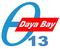TWiki > TWikiVariables > VarCALCULATE Daya Bay webs: Public | 中文 | Internal | Help Log In or Register

### CALCULATE{"formula"} -- handle spreadsheet calculations outside tables

• The `%CALCULATE{formula}%` variable is handled by the SpreadSheetPlugin. Over 100 functions are available, such as `\$ABS()`, `\$EXACT()`, `\$EXISTS()`, `\$GET()/\$SET()`, `\$IF()`, `\$LOG()`, `\$LOWER()`, `\$PERCENTILE()`, `\$TIME()`, `\$VALUE()`.
• Syntax: `%CALC{formula}%`
• Examples:
• `%CALC{\$EXISTS(Web.SomeTopic)}%` returns `1` if the topic exists
• `%CALC{\$UPPER(Collaboration)}%` returns `COLLABORATION`
• Note: The CALCULATE variable is handled inside-out & left-to-right like ordinary TWiki variables, but it does not support functions that refer to table cells, such as `\$LEFT()` or `\$T()`. Use CALC instead.